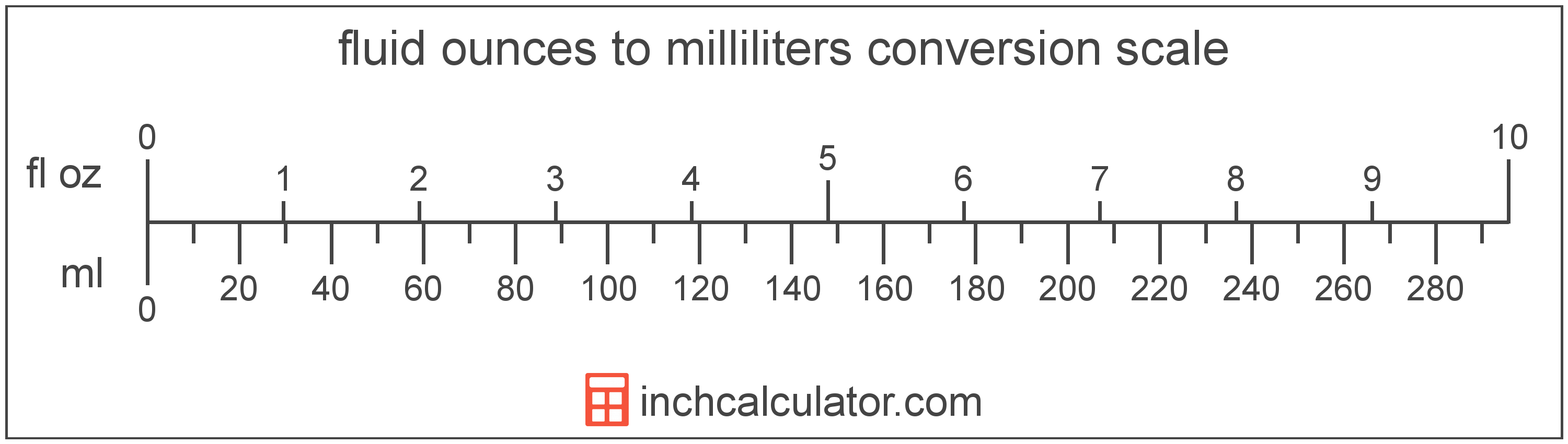# Fluid Ounces to Milliliters Converter

Enter the volume in fluid ounces below to get the value converted to milliliters.

Results in Milliliters:1 fl oz = 29.57353 mL

Do you want to convert milliliters to fluid ounces?

## How to Convert Fluid Ounces to Milliliters

To convert a measurement in fluid ounces to a measurement in milliliters, multiply the volume by the following conversion ratio: 29.57353 milliliters/fluid ounce.

Since one fluid ounce is equal to 29.57353 milliliters, you can use this simple formula to convert:

milliliters = fluid ounces × 29.57353

The volume in milliliters is equal to the volume in fluid ounces multiplied by 29.57353.

For example, here's how to convert 5 fluid ounces to milliliters using the formula above.
milliliters = (5 fl oz × 29.57353) = 147.867648 mL### How Many Milliliters Are in a Fluid Ounce?

There are 29.57353 milliliters in a fluid ounce, which is why we use this value in the formula above.

1 fl oz = 29.57353 mL

## What is a Fluid Ounce?

The US fluid ounce is a unit of volume equal to 1/16 of a pint or 1/8 of a cup. The fluid ounce is sometimes referred to as just an "ounce" but should not be confused with the unit of weight. One fluid ounce is equal to approximately 29.5735 milliliters, but in nutrition labeling, one fluid ounce is rounded to exactly 30 milliliters.

The fluid ounce is a US customary unit of volume. Fluid ounces can be abbreviated as fl oz, and are also sometimes abbreviated as oz fl. For example, 1 fluid ounce can be written as 1 fl oz or 1 oz fl.

## What is a Milliliter?

The milliliter is a unit of volume equal to 1 cubic centimeter, 1/1,000 of a liter, or about 0.061 cubic inches.

The milliliter is an SI unit of volume in the metric system. In the metric system, "milli" is the prefix for thousandths, or 10-3. A milliliter is sometimes also referred to as a millilitre. Milliliters can be abbreviated as mL, and are also sometimes abbreviated as ml or mℓ. For example, 1 milliliter can be written as 1 mL, 1 ml, or 1 mℓ.

Since one milliliter is equivalent to one cubic centimeter, milliliters are sometimes expressed using the abbreviation for a cubic centimeter (cc) for things such as medical dosages or engine displacements.

## Fluid Ounce to Milliliter Conversion Table

Table showing various fluid ounce measurements converted to milliliters.
Fluid Ounces Milliliters
1 fl oz 29.57 mL
2 fl oz 59.15 mL
3 fl oz 88.72 mL
4 fl oz 118.29 mL
5 fl oz 147.87 mL
6 fl oz 177.44 mL
7 fl oz 207.01 mL
8 fl oz 236.59 mL
9 fl oz 266.16 mL
10 fl oz 295.74 mL
11 fl oz 325.31 mL
12 fl oz 354.88 mL
13 fl oz 384.46 mL
14 fl oz 414.03 mL
15 fl oz 443.6 mL
16 fl oz 473.18 mL
17 fl oz 502.75 mL
18 fl oz 532.32 mL
19 fl oz 561.9 mL
20 fl oz 591.47 mL
21 fl oz 621.04 mL
22 fl oz 650.62 mL
23 fl oz 680.19 mL
24 fl oz 709.76 mL
25 fl oz 739.34 mL
26 fl oz 768.91 mL
27 fl oz 798.49 mL
28 fl oz 828.06 mL
29 fl oz 857.63 mL
30 fl oz 887.21 mL
31 fl oz 916.78 mL
32 fl oz 946.35 mL
33 fl oz 975.93 mL
34 fl oz 1,006 mL
35 fl oz 1,035 mL
36 fl oz 1,065 mL
37 fl oz 1,094 mL
38 fl oz 1,124 mL
39 fl oz 1,153 mL
40 fl oz 1,183 mL

## References

1. U.S. Food & Drug Administration, Guidance for Industry: Guidelines for Determining Metric Equivalents of Household Measures, https://www.fda.gov/regulatory-information/search-fda-guidance-documents/guidance-industry-guidelines-determining-metric-equivalents-household-measures
2. National Institute of Standards and Technology, Specifications, Tolerances, and Other Technical Requirements for Weighing and Measuring Devices, Handbook 44 - 2019 Edition, https://nvlpubs.nist.gov/nistpubs/hb/2019/NIST.HB.44-2019.pdf